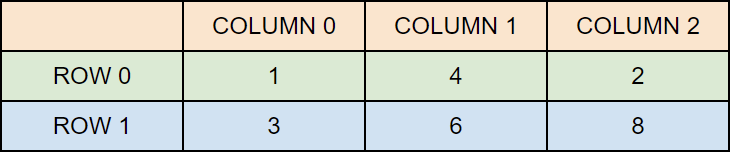# C Multidimensional Arrays

## Multidimensional Arrays

In the previous chapter, you learned about arrays, which is also known as single dimension arrays. These are great, and something you will use a lot while programming in C. However, if you want to store data as a tabular form, like a table with rows and columns, you need to get familiar with multidimensional arrays.

A multidimensional array is basically an array of arrays.

Arrays can have any number of dimensions. In this chapter, we will introduce the most common; two-dimensional arrays (2D).

## Two-Dimensional Arrays

A 2D array is also known as a matrix (a table of rows and columns).

To create a 2D array of integers, take a look at the following example:

int matrix = { {1, 4, 2}, {3, 6, 8} };

The first dimension represents the number of rows , while the second dimension represents the number of columns . The values are placed in row-order, and can be visualized like this:## Access the Elements of a 2D Array

To access an element of a two-dimensional array, you must specify the index number of both the row and column.

This statement accesses the value of the element in the first row (0) and third column (2) of the matrix array.

### Example

int matrix = { {1, 4, 2}, {3, 6, 8} };

printf("%d", matrix);  // Outputs 2
Try it Yourself »

Remember that: Array indexes start with 0:  is the first element.  is the second element, etc.

## Change Elements in a 2D Array

To change the value of an element, refer to the index number of the element in each of the dimensions:

The following example will change the value of the element in the first row (0) and first column (0):

### Example

int matrix = { {1, 4, 2}, {3, 6, 8} };
matrix = 9;

printf("%d", matrix);  // Now outputs 9 instead of 1
Try it Yourself »

## Loop Through a 2D Array

To loop through a multi-dimensional array, you need one loop for each of the array's dimensions.

The following example outputs all elements in the matrix array:

### Example

int matrix = { {1, 4, 2}, {3, 6, 8} };

int i, j;
for (i = 0; i < 2; i++) {
for (j = 0; j < 3; j++) {
printf("%d\n", matrix[i][j]);
}
}
Try it Yourself »

W3Schools is optimized for learning and training. Examples might be simplified to improve reading and learning. Tutorials, references, and examples are constantly reviewed to avoid errors, but we cannot warrant full correctness of all content. While using W3Schools, you agree to have read and accepted our terms of use, cookie and privacy policy.